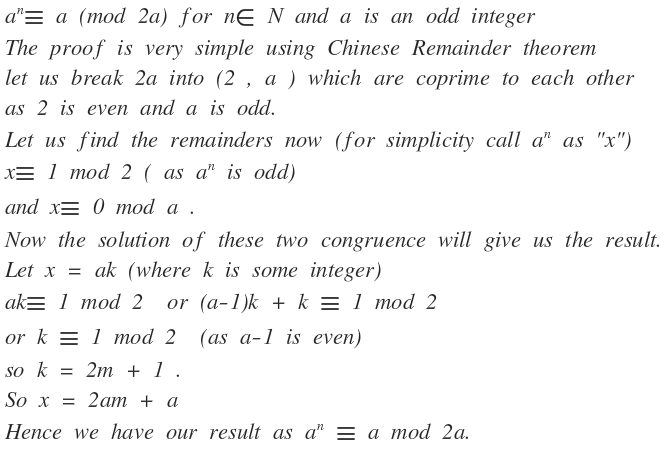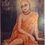# Number Theory Observation

I was doing some number theory problems and while working on a problem I came up with an useful generalization. I know it is not very special but it's worth sharing in my view.1 year ago

This discussion board is a place to discuss our Daily Challenges and the math and science related to those challenges. Explanations are more than just a solution — they should explain the steps and thinking strategies that you used to obtain the solution. Comments should further the discussion of math and science.

When posting on Brilliant:

• Use the emojis to react to an explanation, whether you're congratulating a job well done , or just really confused .
• Ask specific questions about the challenge or the steps in somebody's explanation. Well-posed questions can add a lot to the discussion, but posting "I don't understand!" doesn't help anyone.
• Try to contribute something new to the discussion, whether it is an extension, generalization or other idea related to the challenge.

MarkdownAppears as
*italics* or _italics_ italics
**bold** or __bold__ bold
- bulleted- list
• bulleted
• list
1. numbered2. list
1. numbered
2. list
Note: you must add a full line of space before and after lists for them to show up correctly
paragraph 1paragraph 2

paragraph 1

paragraph 2

[example link](https://brilliant.org)example link
> This is a quote
This is a quote
    # I indented these lines
# 4 spaces, and now they show
# up as a code block.

print "hello world"
# I indented these lines
# 4 spaces, and now they show
# up as a code block.

print "hello world"
MathAppears as
Remember to wrap math in $$ ... $$ or $ ... $ to ensure proper formatting.
2 \times 3 $2 \times 3$
2^{34} $2^{34}$
a_{i-1} $a_{i-1}$
\frac{2}{3} $\frac{2}{3}$
\sqrt{2} $\sqrt{2}$
\sum_{i=1}^3 $\sum_{i=1}^3$
\sin \theta $\sin \theta$
\boxed{123} $\boxed{123}$

Sort by:

Nice result! However, I think you can greatly improve your writing and formatting; it seems very hard to read and follow, but I would probably chalk that down to your possible lack of experience with mathematical writing.

I know but I cannot figure out how to use Latex .(I have absolutely 0 coding experience as I had never taken up computer science.) I try to use it but the writing gets all messed up. Maybe someone will type it out on Latex.

I'm not just talking about LaTeX (I can understand that you may not know how to use it); I found the wording of the proof quite hard to follow. For example, instead of saying lines 2 to 7, you could say the following:

"As $2$ and $a$ are coprime, we would like to find $x \equiv a^n$ (here, the "$\equiv$" sign means "defined to be") such that

$x = 1 \quad (\text{mod } 2)$

and

$x = 0 \quad (\text{mod } a).$

We note that this solution is unique by the Chinese Remainder Theorem."

If you were using LaTeX, you'd also want to take advantage of the Theorem/Proof layout, but even if you don't you would need to separate the theorem from the result. The subsequent six lines could also be rewritten in a similar flavour.

Okay understood. I will try to better it ................You know after we've kicked our asses in cricket :)

I hate the Aussie cricket team. National disgraces.

I prefer rugby myself. I am a huge fan of Dave Pocock and Ben Foley

Somewhat more directly, it just boils down to $a^2 \equiv a \pmod{2a}$. This follows since $a (a-1) \equiv 0 \pmod{2a}$ as the first term is a multiple of $a$ and the second term is a multiple of 2.

Staff - 11 months, 3 weeks ago